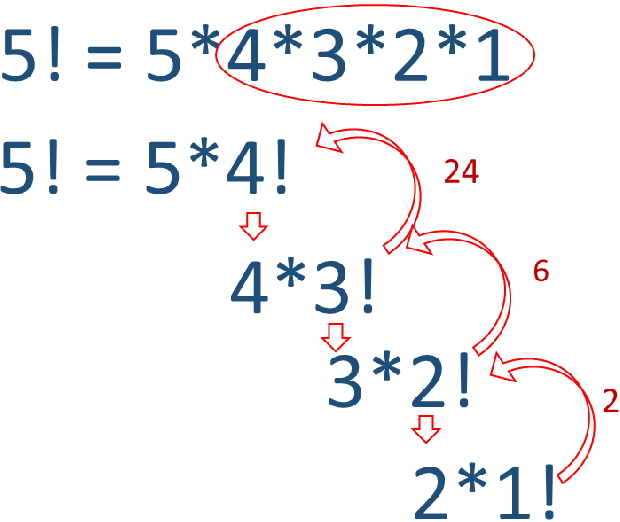# Factorial of a Number using PHP

Factorial of a number is product of all integers from 1 up to the number ( inclusive ).
Factorial of 5 is 1*2*3*4*5 = 120
Or
n! = 1*2*3….n

## Factorial using looping

``````\$n1=50; // change this number
for(\$i=1;\$i<=(\$n1/2); \$i++){
if(\$n1%\$i == 0 )
echo " \$i," ;
}
echo  \$n1 ;``````
Factorial of an input number through sticky form in PHP using for loop or using recursive function

## Using for loop

More details on for loop in PHP.
``````\$n1=5; // change this number
\$factorial=1;
for (\$i=1; \$i<=\$n1;\$i++){
\$factorial=\$factorial*\$i;
}
echo "Factorial of \$n1 : \$factorial";
``````
Output
``Factorial of 5 : 120``

We can use one sticky form to take user input and calculate the factorial of input number.
``````\$n1=\$_POST['n1'];
echo "<form method=POST action=''>
<input type=text name=n1 value='\$n1'>
<input type=submit value=Submit>
</form>";
\$factorial=1;
for (\$i=1; \$i<=\$n1;\$i++){
\$factorial=\$factorial*\$i;
}

echo "Factorial of \$n1 : \$factorial";``````

## Factorial of a number by using recursive functionBy using recursive function we can call the function itself from within the function.
``````function fact(\$n){
if(\$n<>1){
\$factorial = \$n * fact(\$n-1);
return \$factorial;
}else{
return 1;
}
}
echo "Factorial of 6 : ".fact(6); ``````
Output is here
``Factorial of 6 : 720``

Subscribe to our YouTube Channel here

## Subscribe

* indicates required
Subscribe to plus2netplus2net.com